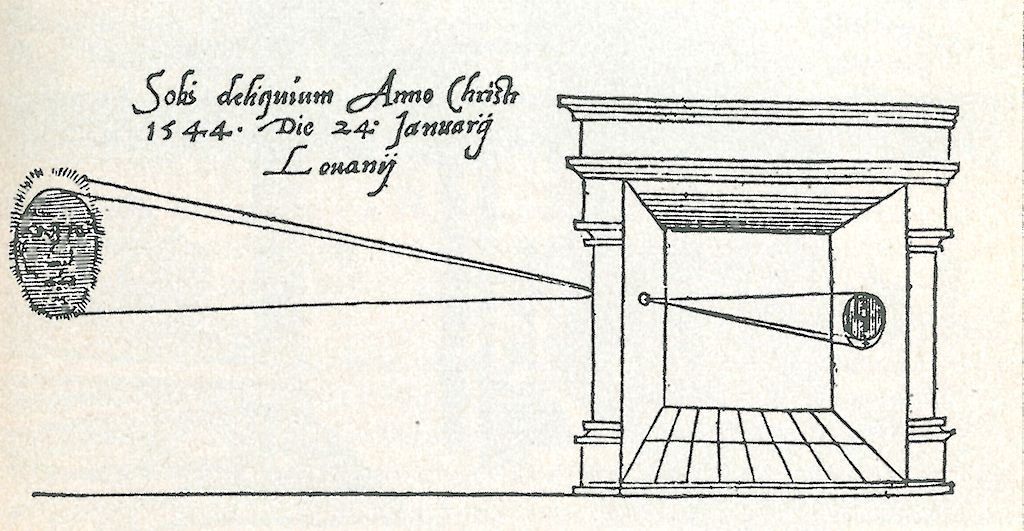# The three main attributes of a camera lens

If you ever want to embark on the foolish pursuit of building a camera, you will need to understand how lenses work. The three attribute that control the behavior of a basic camera lenses are focal length, format and aperture.

## Focal length

The focal length is the distance from the lens at which infinite rays converge. For example, if you have a 50mm lens, infinity is in focus when the plane of the image is 50 millimeters away from the lens. A lens’s focal length is a factor of how much it bends the light. The more the bend, the closer to the lens the rays converge and the smaller the projected image. This, in turn, means that the focal length can also be used as an indication of the magnification of the image.

## Format

The format represents the intended size of the image plane that the lens is designed to project onto, such as 35mm or 4×5. The combination of the focal length and format determine the lens’s field of view. This is why a lens with the same focal length gives you a different amount of magnification on different formats. (illustration)

## Aperture

The aperture gives a measure in f-stops of how “fast” the lens is. It is often thought of as the size of the opening in the lens, but in photography, it actually is the ratio of the focal length over the diameter of the lens opening. This has the advantage of remaining proportionally equal across the different sizes of photographic systems. If you use the same f-stop in a tiny phone camera and a big SLR using the same ISO setting, the same shutter speed will expose both images similarly. As the size of the opening increases, more light gets in but the thinner the focal plane is.

## Finding the focal plane

The last relevant piece of information is the formula that determines the distance of the focal plane to the lens for rays that are closer to the camera than infinity. The relationship between the distance of an object to the lens (S1), and the distance of the lens to the focal plane of that object(S2) is defined by this formula:
`1/S1 + 1/S2 = 1/focal_length`
Solving for S1, this becomes:
` S1 = (S2 * focal_length)/(focal_length - S2)`
If you plug in your own numbers, you will notice that the closer your object is to the lens, the farther away the focal plane will be from the lens.

## Useful python functions

``` # Given a specific focal length and distance to subject, how close to the lens does the image plane converge? def distanceOfFocusPlaneToLens(distanceToSubject, focalLength): v= (focalLength*distanceToSubject)/(distanceToSubject-focalLength) print ("when subject is at %s the focal plane is %s from the lens" % (distanceToSubject, v))```

``` ```

```#FOV calculator def FOVFromFocalAndAperture(focal, aperture):     return math.degrees(2*math.atan(aperture/(focal * 2))) ```